This is archived content, mostly untouched since 2003. For newer content/updated versions, see netfuture.ch.
This is archived content, mostly untouched since 2003. For newer content/updated versions, see netfuture.ch.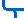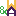Waldvogel & Aschwanden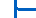Roman PletkaNicola Aschwanden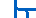Lars Waldvogel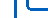Kinderlieder+Spiele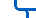Marcel Waldvogel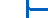ContactPublicationsResearchTutorialsClassesSoftware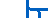Archive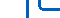Weak Keys in RC4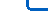Fun

# Weak Keys in RC4

(Original version and the addendum, for both, the PGP signature should verify; printable version)

From: andrewr@vironix.co.za (Andrew Roos)
Newsgroups: sci.crypt
Subject: Weak Keys in RC4
Date: 22 Sep 1995 09:59:45 GMT
Organization: Vironix
Lines: 303
Message-ID: <43u1eh\$1j3@hermes.is.co.za>

```-----BEGIN PGP SIGNED MESSAGE-----
```
A CLASS OF WEAK KEYS IN THE RC4 STREAM CIPHER

PRELIMINARY DRAFT

ANDREW ROOS
VIRONIX SOFTWARE LABORATORIES

## 1. INTRODUCTION

This paper discusses a class of weak keys in RSA's RC4 stream cipher. It shows that for at least 1 out of every 256 possible keys the initial byte of the pseudo-random stream generated by RC4 is strongly correlated with only a few bytes of the key, which effecitively reduces the work required to exhaustively search RC4 key spaces.

## 2. STATE TABLE INITIALIZATION IN RC4

Although the RC4 algorithm has not been published by RSA Data Security, source code to implement the algorithm was anonymously posted to the Cypherpunks mailing list several months ago. The success of the Cypherpunks' brute-force attack on SSL with a 40-bit key indicates that the source code published did accurately implement RC4.

RC4 uses a variable length key from 1 to 256 bytes to initialize a 256-byte state table which is used for the subsequent generation of pseudo-random bytes. The state table is first initialized to the sequence {0,1,2,...,255}. Then:

```1    index1 = 0;
2    index2 = 0;
3
4    for(counter = 0; counter < 256; counter++)
5    {
6        index2 = (key_data_ptr[index1] + state[counter] + index2) % 256;
7        swap_byte(&state[counter], &state[index2]);
8        index1 = (index1 + 1) % key_data_len;
9    }
```

Note that the only line which directly affects the state table is line 7, when two bytes in the table are exchanged. The first byte is indexed by "counter", which is incremented for each iteration of the loop. The second byte is indexed by "index2" which is a function of the key. Hence each element of the state table will be swapped at least once (although possibly with itself), when it is indexed by "counter". It may also be swapped zero, one or more times when it is indexed by "index2". If we assume for the moment that "index2" is a uniformly distributed pseudo-random number, then the probability that a particular single element of the state table will be indexed by "index2" at some time during the initialization routine is:

```     P = 1 - (255/256) ^ 255
= 0.631
```

(The exponent is 255 because we can disregard the case when "index2" and "counter" both index the same element, since this will not affect its value.)

Conversely, there is a 37% probability that a particular element will _not_ be indexed by "index2" during initialization, so its final value in the state table will only be affected by a single swap, when it is indexed by "counter". Since key bytes are used sequentially (starting again at the beginning when the key is exhausted), this implies:

```A.   Given a key length of K bytes, and E < K, there is a 37% probability that
element E of the state table depends only on elements 0..E (inclusive) of
the key.
```

(This is approximate since "index2" is unlikely to be uniformly distributed.)

In order to make use of this, we need to determine the most likely values for elements of the state table. Since each element is swapped at least once (when it is indexed by "counter"), it is necessary to take into account the likely effect of this swap. Swapping is a nasty non-linear process which is hard to analyze. However, when dealing with the first few elements of the state table, there is a high probability that the byte with which the element is swapped has not itself been involved in any previous exchanges, and therefore retains its initial value {0,1,2,...,255}. Similarly, when dealing with the first few elements of the state table, there is also a significant probability that none of the state elements added to index2 in line 6 of the algorithm has been swapped either.

This means that the most likely value of an element in the state table can be estimated by assuming that state[x] == x in the algorithm above. In this case, the algorithm becomes:

```1    index1 = 0;
2    index2 = 0;
3
4    for(counter = 0; counter < 256; counter++)
5    {
6        index2 = (key_data_ptr[index1] + counter + index2) % 256;
7        state[counter] = index2;
8        index1 = (index1 + 1) % key_data_len;
9    }
```

Which can be reduced to:

```B.   The most likely value for element E of the state table is:

S[E] = X(E) + E(E+1)/2
where X(E) is the sum of bytes 0..E (inclusive) of the key.
```

(when calculating the sum of key elements, the key is considered to "wrap around" on itself).

Given this analysis, we can calculate the probability for each element of the state table that it's value is the "most likely value" of B above. The easiest way to do this is to evaluate the state tables produced from a number of pseudo-randomly generated RC4 keys. The following table shows the results for the first 47 elements from a trial of 100 000 eighty-bit RC4 keys:

```          Probability (%)

0-7       37.0  36.8  36.2  35.8  34.9  34.0  33.0  32.2
8-15      30.9  29.8  28.5  27.5  26.0  24.5  22.9  21.6
16-23     20.3  18.9  17.3  16.1  14.7  13.5  12.4  11.2
24-31     10.1   9.0   8.2   7.4   6.4   5.7   5.1   4.4
32-39      3.9   3.5   3.0   2.6   2.3   2.0   1.7   1.4
40-47      1.3   1.2   1.0   0.9   0.8   0.7   0.6   0.6
```

The table confirms that there is a significant correlation between the first few values in the state table and the "likely value" predicted by B.

## 3. WEAK KEYS

The RC4 state table is used to generate a pseudo-random stream which is XORed with the plaintext to give the ciphertext. The algorithm used to generate the stream is as follows:

```     x and y are initialized to 0.

To generate each byte:

1    x = (x + 1) % 256;
2    y = (state[x] + y) % 256;
3    swap_byte(&state[x], &state[y]);
4    xorIndex = (state[x] + state[y]) % 256;
5    GeneratedByte = state[xorIndex];
```

One way to exploit our analysis of the state table is to find circumstances under which one or more generated bytes are strongly correlated with a small subset of the key bytes.

Consider what happens when generating the first byte if state == 1.

```1    x = (0 + 1) % 256;                  /* x == 1 */
2    y = (state + 0) % 256;           /* y == 1 */
3    swap_byte(&state, &state);    /* no effect */
4    xorIndex = (state + state);   /* xorIndex = 2 */
5    GeneratedByte = state
```

And we know that state is has a high probability of being

```     S = K + K + K + 2 (2+1) / 2
```

Similarly,

```     S = K + K + 1 (1+1) / 2
```

So to make it probable that S == 1, we have:

```     K + K == 0 (mod 256)
```

In which case the most likely value for S is:

```     S = K + 3
```

This allows us to identify a class of weak keys:

```C.   Given an RC4 key K..K[N] with K + K == 0 (mod 256), there is a
significant probability that the first byte generated by RC4 will be
K + 3 (mod 256).
```

Note that there are two special cases, caused by "unexpected" swapping during key generation. When K==1, the "expected" output byte is k + 2, and when k==2, the expected value is k + 1.

There are a number of similar classes of "weak keys" which only affect a few keys out of every 65536. However the particular symmetry in this class means that it affects one key in 256, making it the most interesting instance.

Once again I took the easy way out and used simulation to determine the approximate probability that result C holds for any given key. Probabilities ranged between 12% and 16% depending on the values of K and K, with a mean of about 13.8%. All these figures are significantly greater than the 0.39% which would be expected from an uncorrelated generator. The key length used was again 80 bits. This works the other way around as well: given the first byte B generated by a weak key, the probability that K==B-3 (mod 256) is 13.8%.

## 4. EXPLOITING WEAK KEYS IN RC4

Having found a class of weak keys, we need a practical way to attack RC4 based cryptosystems using them. The most obvious way would be to search potential weak keys first during an exhaustive attack. However since only one in every 256 keys is weak, the effective reduction in search space is not particularly significant.

The usefulness of weak keys does increase if the opponent is satisfied with recovering only a percentage of the keys subjected to analysis. Given a known generator output which includes the first generated byte, one could assume that the key was weak and search only the weak keys which would generate the known initial byte. Since 1 in 256 keys is weak, and there is a 13.8% chance that the assumed value of K will be correct, there is only a 0.054% chance of finding the key this way. However, you have reduced the search space by 16 bits due to the assumed relationship between K and K and the assumed value of K, so the work factor per key recovered is reduced by a factor of 35, which is equivalent reducing the effective key length by 5.1 bits.

However in particular circumstances, the known relationships between weak keys may provide a much more significant reduction in workload. The remainder of this section describes an attack which, although requiring very specific conditions, illustrates the potential threat.

As a stream cipher, a particular RC4 key can only be used once. When multiple communications sessions are required, some mechanism must be provided for generating a new session key each time. Let us suppose that an implementation chose the simple method of incrementing the previous session key to get the new session key, and that the session key was treated as a "little endian" (least significant byte first) integer for this purpose.

We now have the interesting situation that the session keys will "cycle through" weak keys in a pattern which repeats every 2^16 keys:

```00 00 00 ...    Weak
(510 non-weak keys)
FF 01 00 ...    Weak
(254 non-weak keys)
FE 02 00 ...    Weak
(254 non-weak keys)
FD 03 00 ...    Weak
...
01 FF 00 ...    Weak
(254 non-weak keys)
00 00 01 ...    Weak
(510 non-weak keys)
FF 01 01 ...    Weak
```

(Least significant byte on the left)

Now while an isolated weak key cannot be identified simply from a known generator output, this cycle of weak keys at known intervals can be identified using statistical techniques since each of the weak keys has a higher than expected probability of generating the _same_ initial byte. This means that an opponent who knew the initial generated bytes of about 2^16 session keys could identify the weak keys, and would also be able to locate the 510-key gap between successive cycles of weak keys (although not precisely). Since the 510-key gap occurs immediately following a key which begins with 00 00, the opponent not only knows that the keys are weak, but also knows the first two bytes of each key. The third byte of each key can be guessed from the first output byte generated by the key, with a 13.8% chance of a correct guess. Assuming that the "510-key gap" is narrowed down to 1 of 8 weak keys, the attacker can search a key space which is 24 bits less than the size of the session keys, with a 13.8%/8 chance of success, effectively reducing the key space by approximately 18 bits.

Although this particular attack depends on a very specific set of circumstances, it is likely that other RC4 based cryptosystems in which there are linear relationships between successive session keys could be vulnerable to similar attacks.

## 5. RECOMMENDATIONS

The attacks described in this algorithm result from inadequate "mixing" of key bytes during the generation of the RC4 state table. The following measures could be taken to strengthen cryptosystems based on the RC4 algorithm:

```(a) After initializing the algorithm, generate and discard a number of bytes.
Since the algorithm used to generate bytes also introduces additional
non-linear dependencies into the state table, this would make analysis
more difficult.

(b) In systems which require multiple session keys, ensure that session keys
are not linearly related to each other.

(c) Avoid using the weak keys described.
```

## 6. CONCLUSION

This preliminary analysis of RC4 shows that the algorithm is vulnerable to analytic attacks based on statistical analysis of its state table. It is likely that a more detailed analysis of the algorithm will reveal more effective ways to exploit the weaknesses described.

Andrew Roos <andrewr@vironix.co.za>
```-----BEGIN PGP SIGNATURE-----
Version: 2.6.2i

iQCVAwUBMGLAk2atuqa4OR+lAQGYJQQA1W2r/giH1iPxeLRjooPEvAJJO2GHrBNy
h1fjHhPf6uBhBapEyZfN5utaUZYkkz/3tXJQC1p+17XwAJHGxb6kapHl3tAf2k5B
P7C034fo8WIOmam1GQqlG3c1MPjCvkNY02NEkYAmNtcwKMP96QgDMCbvS0kn55WE
L1GOWMVYqO4=
=iogI
-----END PGP SIGNATURE-----
```

## One Week Later...

From: andrewr@vironix.co.za (Andrew Roos)
Subject: Cryptanalysis of RC4 - Preliminary Results
Date: 1995/09/28
Message-ID: <44ebge\$llf@hermes.is.co.za>
organization: Vironix-ZA
newsgroups: sci.crypt

```-----BEGIN PGP SIGNED MESSAGE-----
```

Hi c'punks & sci.cryptites

About a week ago I posted a message about weak keys in RC4. This is an update on the results of my continued 4am sessions with RC4 and shows that certain weak keys lead to an almost-feasible known plaintext attack on the cipher (well, about as feasible as the differential attack on DES, shall we say).

The attack is based on two particularly interesting three-byte key prefixes which have a high probability of producing PRNG sequences which start with a known two-byte sequence. The prefixes are:

```1.  Keys starting with "00 00 FD" which have a 14% probability of
generating sequences which start "00 00".

2.  Keys starting with "03 FD FC" which have a 5% probability of
generating sequences which start "FF 03".
```

Note that the expected frequency of any two-byte output sequence is 1 in 65536 or about 0.0015%, so these key prefixes are highly unusual. I won't go into the reasons why in this post, since it follows the same reasoning as my last post, but these prefixes are special in that they have a high probability of initializing the RC4 state table in such a way that the first two generated bytes depend only on the first three entries in the state table.

This observation is the basis for a simple known-plaintext attack which reduces the effective key space which you need to search to have a 50% probability of discovering a key by about 11.2 bits. The down side is that you need "quite a few" known plaintexts to make the attack feasible.

It works as follows:

```1.  Collect a large number of known plaintexts (and hence known
generator sequences).

"FF 03".

3.  For generator streams starting "00 00", search all keys which
begin with "00 00 FD".

4.  For generator streams staring "FF 03", search all keys which
begin with "03 FD FC".

5.  Keep going until you find a key :-)
```

Clearly this attack will only discover a small fraction of the keys. However since most generator sequences are discarded without being searched, and for those which are searched the search is 2^24 smaller than would be required to search the entire keyspace, the number of trials required to determine a key is significantly lower than for brute force alone.

Enough of an intro, here are the relevant results. Forgive my simplistic approach to maths, I'm a philosopher-come-software developer, not a mathematician. I've run the relevant simulations with 40-bit, 64-bit, 80-bit and 128-bit key lengths, and with two different PRNGs. For the sake of consistency with my earlier paper I'll use the figures gathered for 80-bit keys (this seems to be RSA's preferred key length for RC4), but there are no significant differences for other key lengths. The PRNG used for these tests was L'Ecuyer's 32-bit combined linear congruential generator as described in "Applied Cryptography" p. 349.

```(a) Out of one million trials, keys starting with "00 00 FD"
generated sequences starting "00 00" 138217 times, and keys
starting with "03 FD FC" generated output sequences starting "FF
03" 50490 times.

(b) Out of ten million trials, arbitrary pseudo-random keys generated
sequences starting with "00 00" 446 times, and sequences starting
with "FF 03" 146 times. (Note the abnormally high incidence of
"00 00"; the expected mean is 152.8).
```

Suppose we have the output stream generated by a randomly chosen key. The chance that it will start with either "00 00" or "FF 03", and that we will therefore search it, is:

```    (446 + 146) / 1e7 = 5.92e-5
```

The chance that it starts with "00 00" and was generated by a key starting with "00 00 FD", or that it starts with "FF 03" and was generated by a key starting "03 FD FC" - i.e. the chance that we will search it and be rewarded for our efforts - is:

```    (138217 + 50490)/(1e6 * 2^24) = 1.12e-8
```

The total number of plaintexts required for a 50% chance that we will discover one of the keys is:

```    log(0.5)/log(1 - 1.12e-8) = 61 900 000
```

Well I did say "quite a few" plaintexts would be necessary :-)

And the number of plaintexts which you expect to search in order to find the "right" one is:

```    61 900 000 * 5.92e-5 = 3665
```

Since the total key length is 80 bits, and we are "guessing" 24 of these, each search requires 2^56 trials. Hence the total number of trials for a 50% chance of discovering a key is:

```    3665 * 2^56 = 2.64e20 = 2 ^ 67.8
```

Since brute search alone would require 2^79 trials for a 50% chance of determining the key, this reduces the number of trials by 2^11.2.

The results are essentially identical for all the key lengths I have tried, and in each case reduce effective key length by about 11.2 bits. So, for example, a 64-bit key would normally require 2^63 trials for 50% chance of solution; this attack reduces the number of trials to 2^51.8 at the cost of requiring 62 million known plaintexts.

I'm still running simulations to check my maths, and although initial results are encouraging, I don't have enough data for it to be statistically relevant yet (generating all these sets of 62 million known streams takes time...) So consider this preliminary (again), and I'll post the results of my simulations when I have enough data.

Andrew
```________________________________________________________________
Andrew Roos

// C++ programmers have class (but not much inheritance)

PGP Fingerprint: F6 D4 04 6E 4E 16 80 59 3A F2 27 94 8B 9F 40 26
Full key at ftp://ftp.vironix.co.za/PGP-keys/AndrewRoos

-----BEGIN PGP SIGNATURE-----
Version: 2.6.2i

iQCVAwUBMGrlfmatuqa4OR+lAQF1eQP+IBBmSztAYUpq1q/BjzvYDCbb+Ns0Gi1S
u9wTaZOCl32fdp7NSUEQBX39nVJkQZginug56BZXzijRvOx6fl4+z7dmW9jwtE5E
YNCOhx+/fHX4psszMyEUTrnza7MYDc4HXlgv743LOD/xvEyU0D5OGgB5fg+lyhAK
6xQ/Zy8JpE8=
=BdMn
-----END PGP SIGNATURE-----
```